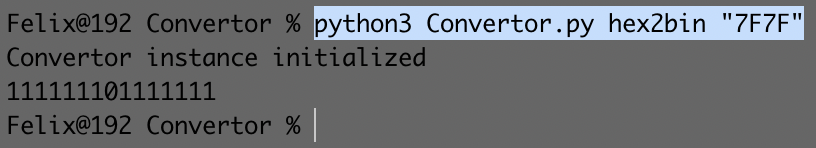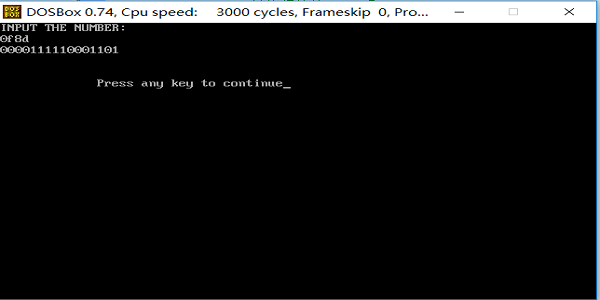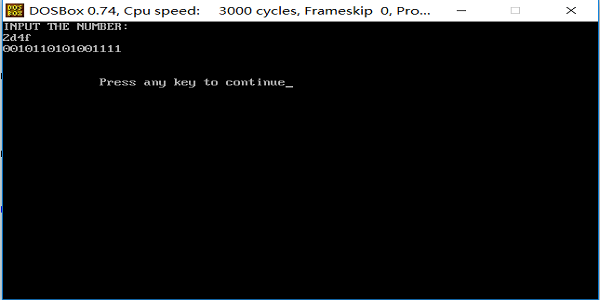• 在解决该题之前，我们先来了解一下十进制转换二进制的方法将十进制转换二进制的方法方法一：利用Interger.toBinaryString()的方法。方法二：除基倒取余法 输入一个十进制数n，每次用n除以2，把余数记下来，再用...
在解决该题之前，我们先来了解一下十进制转换成二进制的方法将十进制转换成二进制的方法方法一：利用Interger.toBinaryString()的方法。方法二：除基倒取余法       输入一个十进制数n，每次用n除以2，把余数记下来，再用商去除以2...依次循环，直到商为0结束，把余数倒着依次排列，就构成了转换后的二进制数public class DecimalToBinary {

public static int decimalToBinary(int n){
//存余数
int r = 0;
//记录所求的二进制数
int bin = 0;
//记录位数
int t = 0;
while (n != 0) {
r = n % 2;
n = n / 2;
bin += (int) (r * Math.pow(10, t));
t++;
}
return bin;
}
public static void main(String[] args) {
int n1 = -9;
int n2 = 10;
int n3 = 1023;
int n4 = 1024;
int n5 = -1023;
int n6 = -1024;
System.out.println("十进制数"+n1+"所对应的二进制数为："+decimalToBinary(n1));
System.out.println("十进制数"+n2+"所对应的二进制数为："+decimalToBinary(n2));
System.out.println("十进制数"+n3+"所对应的二进制数为："+decimalToBinary(n3));
System.out.println("十进制数"+n4+"所对应的二进制数为："+decimalToBinary(n4));
System.out.println("十进制数"+n5+"所对应的二进制数为："+decimalToBinary(n5));
System.out.println("十进制数"+n6+"所对应的二进制数为："+decimalToBinary(n6));
}
}结果：十进制数-9所对应的二进制数为：-1001
十进制数10所对应的二进制数为：1010
十进制数1023所对应的二进制数为：1111111111
十进制数1024所对应的二进制数为：2147483647
十进制数-1023所对应的二进制数为：-1111111111
十进制数-1024所对应的二进制数为：-2147483648从结果可以看出，该程序不能表示负数，且-1023到1023之间的十进制数可以用二进制表示，但是超过了1024就不行了。为什么超过1024就不能表示了呢？因为这个程序我们表示二进制数的时候，我们用的依旧是十进制的形式，大家知道int的取值范围是-2147483648~2147483647.根据我们这个程序，1024所对应的二进制数为10000000000（注意：这个使用的十进制的形式），它超过了int的取值范围。所以它就会转换为一个其他的数字。但是为什么会是这个数字呢？方法三：在方法二的基础上使用字符串对结果集进行存储public class DecimalToBinary {
public static String decimalToBinary(int n){
String str = "";
while (n != 0) {
str = n % 2 + str;
n = n / 2;
}
return str;
}
public static void main(String[] args) {
int n1 = -9;
int n2 = 10;
int n3 = 1023;
int n4 = 1024;
int n5 = -1023;
int n6 = -1024;
int n7 = 1025;
System.out.println("十进制数"+n1+"所对应的二进制数为："+decimalToBinary(n1));
System.out.println("十进制数"+n2+"所对应的二进制数为："+decimalToBinary(n2));
System.out.println("十进制数"+n3+"所对应的二进制数为："+decimalToBinary(n3));
System.out.println("十进制数"+n4+"所对应的二进制数为："+decimalToBinary(n4));
System.out.println("十进制数"+n5+"所对应的二进制数为："+decimalToBinary(n5));
System.out.println("十进制数"+n6+"所对应的二进制数为："+decimalToBinary(n6));
System.out.println("十进制数"+n7+"所对应的二进制数为："+decimalToBinary(n7));
}
}结果：十进制数-9所对应的二进制数为：-100-1
十进制数10所对应的二进制数为：1010
十进制数1023所对应的二进制数为：1111111111
十进制数1024所对应的二进制数为：10000000000
十进制数-1023所对应的二进制数为：-1-1-1-1-1-1-1-1-1-1
十进制数-1024所对应的二进制数为：-10000000000
十进制数1025所对应的二进制数为：10000000001不足：这种方法只能处理正数方法三改进：public static String decimalToBinary(int n){
String result = "";
boolean minus = false;

//如果该数字为负数，那么进行该负数+1之后的绝对值的二进制码的对应位取反，然后将它保存在result结果中
if(n < 0){
minus = true;
n = Math.abs(n + 1);
}

while(true){
int remainder = (!minus && n % 2 == 0) || (minus && n % 2 == 1) ? 0 : 1;

//将余数保存在结果中
result = remainder + result;
n /= 2;

if(n == 0){
break;
}
}

//判断是否为负数，如果是负数，那么前面所有位补1
if(minus){
n = result.length();
for(int i = 1; i <= 32 - n; i++){
result = 1 + result;
}
}
return result;
}
public static void main(String[] args) {
int n1 = -1;
int n2 = 10;
int n3 = 1023;
int n4 = 1024;
int n5 = -1023;
int n6 = -1024;
int n7 = 1025;
System.out.println("十进制数"+n1+"所对应的二进制数为："+decimalToBinary(n1));
System.out.println("十进制数"+n2+"所对应的二进制数为："+decimalToBinary(n2));
System.out.println("十进制数"+n3+"所对应的二进制数为："+decimalToBinary(n3));
System.out.println("十进制数"+n4+"所对应的二进制数为："+decimalToBinary(n4));
System.out.println("十进制数"+n5+"所对应的二进制数为："+decimalToBinary(n5));
System.out.println("十进制数"+n6+"所对应的二进制数为："+decimalToBinary(n6));
System.out.println("十进制数"+n7+"所对应的二进制数为："+decimalToBinary(n7));
}结果：十进制数-1所对应的二进制数为：11111111111111111111111111111111
十进制数10所对应的二进制数为：1010
十进制数1023所对应的二进制数为：1111111111
十进制数1024所对应的二进制数为：10000000000
十进制数-1023所对应的二进制数为：11111111111111111111110000000001
十进制数-1024所对应的二进制数为：11111111111111111111110000000000
十进制数1025所对应的二进制数为：10000000001方法四：利用“移位”public static String decimalToBinary(int n){
String str = "";
for(int i = 31; i >= 0; i--){
int s = n >>> i & 1;
str = str + s;
}
return str;
}
public static void main(String[] args) {
int n1 = -1;
int n2 = 10;
int n3 = 1023;
int n4 = 1024;
int n5 = -1023;
int n6 = -1024;
int n7 = 1025;
System.out.println("十进制数"+n1+"所对应的二进制数为："+decimalToBinary(n1));
System.out.println("十进制数"+n2+"所对应的二进制数为："+decimalToBinary(n2));
System.out.println("十进制数"+n3+"所对应的二进制数为："+decimalToBinary(n3));
System.out.println("十进制数"+n4+"所对应的二进制数为："+decimalToBinary(n4));
System.out.println("十进制数"+n5+"所对应的二进制数为："+decimalToBinary(n5));
System.out.println("十进制数"+n6+"所对应的二进制数为："+decimalToBinary(n6));
System.out.println("十进制数"+n7+"所对应的二进制数为："+decimalToBinary(n7));
}结果：十进制数-1所对应的二进制数为：11111111111111111111111111111111
十进制数10所对应的二进制数为：00000000000000000000000000001010
十进制数1023所对应的二进制数为：00000000000000000000001111111111
十进制数1024所对应的二进制数为：00000000000000000000010000000000
十进制数-1023所对应的二进制数为：11111111111111111111110000000001
十进制数-1024所对应的二进制数为：11111111111111111111110000000000
十进制数1025所对应的二进制数为：00000000000000000000010000000001说明：由于计算机中存储的都是数的补码，正数的原码、反码、补码都是相同的；而负数的原码、反码、补码是不一样的，补码=原码取反+1（符号位不变）。所以，负数是按照它的补码输出的。>>>为逻辑移位符，向右移n位，高位补0>> 算数移位符，也是向右移n位，不同的是：正数高位补0，负数高位补1<< 移位符，向左移n位，低位补0二进制中1的个数题目描述：输入一个整数，输出该数二进制表示中1的个数。其中负数用补码表示。分析：先将十进制的数转换成二进制数，再进行判断解法一：将字符串转成字符数组的形式，遍历进行判断public static int NumberOf1(int n) {
String result = Integer.toBinaryString(n);
System.out.println("n的二进制数："+result);
//将字符串转换成字符数组，进行判断
char[] res = result.toCharArray();
int count = 0;
System.out.println("二进制位数："+res.length);
for(int i = 0;i < res.length; i++){
if (res[i] == '1') {
count ++;
}
}
return count;
}

public static void main(String[] args) {
Scanner scanner = new Scanner(System.in);
System.out.print("请输入一个数字n:");
int n = scanner.nextInt();
System.out.println(n+"的二进制数中表示1的个数为："+NumberOf1(n));
}测试结果：请输入一个数字n:4
n的二进制数：100
二进制位数：3
4的二进制数中表示1的个数为：1请输入一个数字n:-3
n的二进制数：11111111111111111111111111111101
二进制位数：32
-3的二进制数中表示1的个数为：31建议：在上述方法中，我们是将二进制数直接用字符串输出；其实也可以利用Interger.parseInt()转化成int。但是不建议用这种方式，我试了一下，当输入数字为-3时，它的二进制数应该为11111111111111111111111111111101 ，但是此时出现了java.lang.NumberFormatException错误。这是为什么呢？因为超过了int类型表示的范围.解法二：Integer.toBinaryString(n).replace("0","").length();public static int NumberOf11(int n) {
return Integer.toBinaryString(n).replace("0", "").length();
}解法三：利用&public static int NumberOf1(int n) {
int count = 0;
while (n != 0) {
count++;
n = n & (n-1);

}
return count;
}如果没有看懂的话，我们来举个例子假如 n = 5  count = 0
n          0101   5
n - 1      0100   4
n=n&(n-1)  0100   4   count = 1
n - 1      0011   3
n=n&(n-1)  0000   0   count = 2
进行了两次&运算，得到二进制5中的1的个数为2延伸：& 按位与  & 既是位运算符又是逻辑运算符，&的两侧可以是int，也可以是boolean表达式，当&两侧是int时，要先把运算符两侧的数转化为二进制数再进行运算。若两个数都为1则为1，否则为0
展开全文剑指offer
• ASCII 全称为 ( American Standard Code for Information Interchange)，简单的说，就是用 7 位二进制 ( 即 十进制表示为 0 到 127 ) (即 十六进制0x00–0x7F) 去编码我们生活中常见的数字，大小写字母，标点
Python3 进制转换(二进制 十进制 十六进制 ASCII字符串转换)
Python3 进制转换
编写Convertor类，用于进制与ASCII字符之间互相转换，便于使用
ASCII码及进制之间转换关系
ASCII码介绍：
ASCII 全称为 ( American Standard Code for Information Interchange)，简单的说，就是用 7 位二进制 ( 即 十进制表示为 0 到 127 ) (即 十六进制0x00–0x7F) 去编码我们生活中常见的数字，大小写字母，标点符号以及一些特殊的控制字符，如下：

数字：0 , 1 , 2 , 3 … 9
字母：a , b , c …z , A , B , C … Z
标点符号以及运算符：，. + - …
控制字符：回车，换行等控制字符

Convertor类的使用：
#!/usr/local/bin/env python3
import struct, sys

base = [str(x) for x in range(10)] + [chr(x) for x in range(ord('A'), ord('A') + 6)]

'''
int(x [,base ])         将x转换为一个整数
long(x [,base ])        将x转换为一个长整数
float(x )               将x转换到一个浮点数
complex(real [,imag ])  创建一个复数
str(x )                 将对象 x 转换为字符串
repr(x )                将对象 x 转换为表达式字符串
eval(str )              用来计算在字符串中的有效Python表达式,并返回一个对象
tuple(s )               将序列 s 转换为一个元组
list(s )                将序列 s 转换为一个列表
chr(x )                 将一个整数转换为一个字符
unichr(x )              将一个整数转换为Unicode字符
ord(x )                 将一个字符转换为它的整数值
hex(x )                 将一个整数转换为一个十六进制字符串
oct(x )                 将一个整数转换为一个八进制字符串
'''

class Convertor(object):

def __init__(self):
self.base = base
# self.base = ['0', '1', '2', '3', '4', '5', '6', '7', '8', '9', 'A', 'B', 'C', 'D', 'E', 'F']
print("Convertor instance initialized")

# Todo: binary to decimal
# eg: bin2dec("00110010") => 50
def bin2dec(self, string_num):
return int(string_num, 2)

# Todo: binary to hex
# eg: bin2hex("1110") => E
def bin2hex(self, string_num):
return self.dec2hex(self.bin2dec(string_num))

# Todo: decimal to binary
# eg: dec2bin(2) => 10
def dec2bin(self, string_num):
num = int(string_num)
mid = []
while True:
if num == 0: break
num, rem = divmod(num, 2)
mid.append(self.base[rem])
return ''.join([str(x) for x in mid[::-1]])

# Todo: decimal to hex
# eg: dec2hex(100) => 64
def dec2hex(self, string_num):
num = int(string_num)
mid = []
while True:
if num == 0: break
num, rem = divmod(num, 16)
mid.append(self.base[rem])

return ''.join([str(x) for x in mid[::-1]])

# Todo: hex to binary
# eg: hex2bin("DF215678ff") => 1101111100100001010101100111100011111111
def hex2bin(self, string_num):
return self.dec2bin(self.hex2dec(string_num.upper()))

# Todo: hex to decimal
# eg: hex2dec("FF") => 255
def hex2dec(szelf, string_num):
return int(string_num.upper(), 16)

# Todo: hex(00--7F) to ASCII
# eg: hex2ASCII("56 65 76 44") => VevD
#     hex2ASCII("56657644") => VevD
def hex2ASCII(self, string_hex, littleEndian=False):
hexStr = (string_hex.strip()).replace(" ", "")
if littleEndian == True:
hexStr = self.littleEndian(hexStr)
asciiVal = bytes.fromhex(hexStr).decode("ASCII")
return asciiVal

# Todo: hex(00--FF) to float
# eg: hex2float("40 36 2A A3") => 2.846352338791
#     hex2float("A3 2A 36 40", littleEndian=True) => 2.846352338791
def hex2float(self,target_num, littleEndian=False):
target_num = target_num.replace(" ", "")
if littleEndian == True:
if target_num.startswith("0x"):
target_num = "0x{}".format(self.littleEndian(target_num[2::]))
else:
target_num = "0x" + self.littleEndian(target_num)
try:
if "0x" in target_num:
target_num = target_num.split("0x")
if " " in target_num:
target_num = target_num.replace(" ", "")
hex1 = bytes.fromhex(target_num)
res = struct.unpack("!f", hex1)
except Exception as e:
print("\033[1;31m{}\033[0m".format(e))
res = 0
return float(res)

# Todo: little endian for hex string value
# eg:   littleEndian("56 77 43") => "437756"
def littleEndian(self, hexStr):
if len(hexStr) %2 !=0:
raise Exception("non-hexadecimal number found in \"{}\"".format(hexStr))
littleEndianHex = ""
for i in range(len(hexStr) - 1, -1, -1):
if i % 2 == 0:
littleEndianHex = "{}{}{}".format(littleEndianHex, hexStr[i], hexStr[i + 1])
hexStr = littleEndianHex
return hexStr

使用说明：

二进制转换：
bin2dec(bin_val)及bin2hex(binary)转换参数为字符串如: “0100”/“10”/“10001100”
十进制转换:
dec2bin(decimal)及dec2hex(decimal)参数decimal类型需要为整数;
十六进制转换:
hex2bin(hexVar)参数需要为十六进制字符,不允许长度为单数,eg:“67DFC2”;
hex2dec(hexVar,littleEndian=False), 参数字符串hexVar在方法内会先进行trim空行空格和删除空格的动作,对处理过的字符进行转换,littleEndian如为True,会对字符串进行littleEndian动作,如"47657F" => “7F6547”;
hex2ASCII(string_hex, littleEndian=False)将十六进制字符串**(00–7F)**转换成ASCII字符串; 十六进制字符80–FF是non-ASCII字符,不能转换成ASCII字符
hex2float(target_num, littleEndian=False)将十六进制字符转换为float
littleEndian(hexStr)可以将十六进制字符串进行倒置"57697F" => “7F6957”

使用案例：
主程式:
#!/usr/local/bin/env python3

from Convertor import Convertor

if __name__ == "__main__":
convertor = Convertor()

# binary to decimal
bin_val = "00110010"
decimal = convertor.bin2dec(bin_val)
print("bin2dec:%d"%decimal)

# binary to hex
binary = "1110"
hexVal = convertor.bin2hex(binary)
print("bin2hex:%s"%hexVal)

# decimal to bin
decimal = 2
binVal  = convertor.dec2bin(decimal)
print("dec2bin:%s"%binVal)

# decimal to hex
decimal = 100
hexVal = convertor.dec2hex(decimal)
print("dec2hex:%s"%hexVal)

# hex to binary
hexVar = "DF215678ff"
binVal = convertor.hex2bin(hexVar)
print("hex2bin:%s"%binVal)

# hex to decimal
hexVar = "FF"
decimalVal = convertor.hex2dec(hexVar)
print("hex2dec:%d"%decimalVal)

# hex string to ASCII
hexStr = "56 65 76 44"
asciiVal = convertor.hex2ASCII(hexStr)
asciiLittleEndianVal = convertor.hex2ASCII(hexStr,littleEndian=True)
print("hex2ASCII:%s\nasciiLittleEndianVal:%s"%(asciiVal,asciiLittleEndianVal))

# hex to float
hexStr = "40 36 2A A3"
floatVal = convertor.hex2float(hexStr)
print("float value:%.12f"%floatVal)

输出:
Convertor instance initialized
bin2dec:50
bin2hex:E
dec2bin:10
dec2hex:64
hex2bin:1101111100100001010101100111100011111111
hex2dec:255
hex2ASCII:VevD
asciiLittleEndianVal:DveV
float value:2.846352338791

通过外部函数名字符串,调用Convertor类的对应方法进行进制转换
代码:
if __name__ == "__main__":
convertor = Convertor()
functionPointer = getattr(convertor, sys.argv)
retVal = None
if len(sys.argv) >= 4 and sys.argv == "littleEndian":
try:
retVal = functionPointer(sys.argv, littleEndian=True)
except Exception as e:
print("\033[1;31m{}\033[0m".format(e))
retVal = functionPointer(sys.argv)
else:
retVal = functionPointer(sys.argv)
print(retVal)

命令行参数执行:
python3 Convertor.py hex2bin "7F7F"展开全文• C# 进制转换二进制、十六进制、十进制互转） 由于二进制数在C#中无法直接表示，所以所有二进制数都用一个字符串来表示 例如： 二进制： 1010 表示为 字符串：“1010” int d = 10; //十进制转二进制字符串 Console...
C# 进制转换（二进制、十六进制、十进制互转）
由于二进制数在C#中无法直接表示，所以所有二进制数都用一个字符串来表示
例如： 二进制： 1010 表示为 字符串：“1010”
int d = 10;
//十进制转二进制字符串
Console.WriteLine(Convert.ToString(d,2));
//输出: 1010
//十进制转十六进制字符串
Console.WriteLine(Convert.ToString(d,16));
//输出: a
//二进制字符串转十进制数
string bin = “1010”;
Console.WriteLine(Convert.ToInt32(bin,2));
//输出: 10
//二进制字符串转十六进制数
string bin = “1010”;
Console.WriteLine(string.Format("{0:x}",Convert.ToInt32(bin,2));
//输出: a
//十六进制转二进制字符串
Console.WriteLine(Convert.ToString(0xa,2));
//输出: 1010
//十六进制转十进制数
Console.WriteLine(Convert.ToString(0xa,10));
//输出: 10
C#实现转换十六进制
C#语言有很多值得学习的地方，这里我们主要介绍C#实现转换十六进制，包括介绍用来表示十六进制的枚举值是 HexNumber等方面。
C#实现转换十六进制
任何数据在计算机内部都是以二进制保存的，所以进制与数据的存储无关，只与输入输出有关。所以，对于进制转换，我们只关心字符串中的结果。
在上面的第 4 条中提到了 ToString() 方法可以将数值转换成字符串，不过在字符串中，结果是以十进制显示的。现在我们带给它加一些参数，就可以让C#实现转换成十六进制——使用 ToString(string) 方法。
这里需要一个 string 类型的参数，这就是格式说明符。十六进制的格式说明符是 “x” 或者 “X”，使用这两种格式说明符的区别主要在于 A-F 六个数字：“x” 代表 a-f 使用小写字母表示，而 “X” 而表示 A-F 使用大字字母表示。如下例：

private void TestHex() {
int a = 188;
this.textBox1.Text = “”;
this.textBox1.AppendText("a(10) = " + a.ToString() + “\n”);
this.textBox1.AppendText("a(16) = " + a.ToString(“x”) + “\n”);
this.textBox1.AppendText("a(16) = " + a.ToString(“X”) + “\n”);
}

运行结果如下：

a(10) = 188
a(16) = bc
a(16) = BC

这时候，我们可能有另一种需求，即为了显示结果的整齐，我们需要控制十六进制表示的长度，如果长度不够，用前导的 0 填补。解决这个问题，我们只需要在格式说明符“x”或者“X”后写上表示长度的数字就行了。比如，要限制在 4 个字符的长度，可以写成“X4”。在上例中追加一句：

this.textBox1.AppendText("a(16) = " + a.ToString(“X4”) + “\n”);

其结果将输出 a(16) = 00BC。
现在，我们还要说一说如何将一个表示十六进制数的字符串转换成整型。这一转换，同样需要借助于 Parse() 方法。这里，我需要 Parse(string, System.Globalization.NumberStyles) 方法。第一个参数是表示十六进制数的字符串，如“AB”、“20”(表示十进制的 32) 等。第二个参数 System.Globalization.NumberStyles 是一个枚举类型，用来表示十六进制的枚举值是 HexNumber。因此，如果我们要将“AB”转换成整型，就应该这样写：int b = int.Parse(“AB”, System.Globalization.NumberStyles.HexNumber)，最后得到的 b 的值是 171。


展开全文• ## 汇编语言 十六进制转换为二进制

万次阅读 多人点赞 2016-12-03 00:21:44
汇编语言程序，十六进制转换二进制


今天写了十六进制转换为二进制的汇编语言小程序，感觉挺有逻辑性的，运用条件分支，进行转换，这里把自己的心得分享一下，希望对大家有点小小的启示

由于，代码中已经有了详细注释，在这里我只是说出我写该程序的思路，首先得有一个流程图，比自己的想法用流程图表示出来，之后便可以照着流程图将自己的想法用汇编语言实现出来，在不懂得地方，只需要拿笔比划一下运行过程，想想程序怎么运行，我想会得到你想要的答案！！！（说明一下，如果输入错误程序直接结束，未返回提示信息！！！我自己蠢）
DATAS SEGMENT
TIP DB 'INPUT THE NUMBER: ',0AH,0DH,'\$'
DATAS ENDS

STACKS SEGMENT
;此处输入堆栈段代码
STACKS ENDS

CODES SEGMENT
ASSUME CS:CODES,DS:DATAS,SS:STACKS
START:
MOV AX,DATAS
MOV DS,AX

MOV DX,OFFSET TIP ;输出提示输入数字标语
MOV AH,09H
INT 21H

MOV CH,4 ;记录输入数字次数
MOV CL,4 ;一次左移位数
MOV BX,0 ;记录左移之后的数字
MOV DH,16 ;输出十六位二进制数字控制

INPUT:
MOV AH,1
INT 21H
;判断是否在0-9之间
cmp AL,30H
JB EXIT ;比0小则退出
CMP AL,39H
JA COMPER1 ;比9大则判断与A的大小
;若进行到下一步则说明这次输入的是0-9之间的数字，转换成二进制
JMP CHANGE1
COMPER1:
CMP AL,41H ;判断与A的大小
JB EXIT ;若小于A则退出
CMP AL,46H ;判断与F的大小
JA COMPER2 ;若比F大则判断与a的大小
JMP CHANGE2 ;进行到这一步则说明输入的是A-F，转换成二进制
COMPER2:
CMP AL,61H ;判断与a的大小
JB EXIT ;小于a则退出
CMP AL,66H ; 判断与f的大小
JA EXIT ; 大于f则退出
JMP CHANGE3 ; 进行到这一步说明输入的是a-f，转换成二进制

CHANGE1:
SUB AL,30H ;转换成0-9
SHL BX,CL ;左移四位
OR BL,AL ; 进行或运算得到四位二进制
DEC CH ; 输入数字次数自减1
JZ ENT ; 四位十六进制全部输入完毕则打印，否则继续输入
JMP INPUT
CHANGE2:
SUB AL,37H ;此时AL中是A-F，减去37H是0AH，0BH...0FH
SHL BX,CL
OR BL,AL
DEC CH
JZ ENT
JMP INPUT
CHANGE3:
SUB AL,57H ;此时AL中是a-f，减去57H就是0AH，0BH...0FH
SHL BX,CL
OR BL,AL
DEC CH
JZ ENT
JMP INPUT
;所有的CHANGE1，2，3道理都是一样的
ENT:
MOV DL, 0DH ;0D是回车符的ASCII码.
MOV AH, 02H ;输出单个字符.
INT 21H ;输出.
MOV DL, 0AH ;0A是换行符的ASCII码.
MOV AH, 02H
INT 21H
;这里0DH，和0AH要一起使用，单独使用会报错
JMP PRINT
PRINT:
SHL BX,1
JNC L1 ;判断进位，如果是0 跳到了L1
MOV DL,'1' ;输出1
MOV AH,02H
INT 21H
DEC DH
JZ EXIT
JMP PRINT
L1:
MOV DL,'0' ;输出0
MOV AH,02H
INT 21H
DEC DH
JZ EXIT
JMP PRINT
EXIT:
MOV AH,4CH
INT 21H
CODES ENDS
END START这里是运行的结果截图：展开全文• C# 进制转换二进制、十六进制、十进制互转） 由于二进制数在C#中无法直接表示，所以所有二进制数都用一个字符串来表示 例如： 二进制： 1010 表示为 字符串："1010" int d = 10; //十进制转二进制字符串 Console....
• C# 进制转换二进制、十六进制、十进制互转） 由于二进制数在C#中无法直接表示，所以所有二进制数都用一个字符串来表示 例如： 二进制： 1010 表示为 字符串："1010"  int d = 10; //十进制转二进制字符串...C#
• 大家都知道，C语言有自带的十六进制八进制输入输出的格式转换符（我估计大家也不会用）。但是，当题目给出的数很大，超出了long long 的范围，这时候该...不多说了，直接说：因为16是2的四次方，所以直接把二进制的4位
•   （该版课程的内容更新及订正均已停止）  旧版课程打包下载 ----------------------------------... 6.2 二、八、十六进制数转换到十进制数  6.2.1 二进制转换为十进制数<brc 语言
• 这是最符合我们平时的数学逻辑思维的，即输入一个十进制数n，每次用n除以2，把余数记下来，再用商去除以2…依次循环，直到商为0结束，把余数倒着依次排列，就构成了转换后的二进制数。 那么，在实际实现中，可以用...
• 1. 计算机进制转换二进制、八进制、十进制、十六进制互转     二进制的基数为 2，权为2^（2的N次方） 1=1 X 2º 10 = 1 X 2¹ 100= 1 x 2 ²   16进制   41（16进制）转换成 2 进制 ...
• 二进制，八进制，十进制，十六进制之间的关系是什么?浮点数是什么回事？ 本文内容参考自王达老师的《深入理解计算机网络》一书&amp;amp;amp;amp;amp;amp;amp;amp;amp;lt;中国水利水电出版社&amp;amp;amp;amp...
• 定点数    定点数就是指小数点的位置固定不变，小数点的位置通常有两...在浮点表示法中，阶码通常为带符号的纯整数，尾数为带符号的纯小数，浮点灵敏的表示格式如下：    阶 | 阶码
• （原MyBlog）前要说明在我们学习程序设计的过程中，总是会遇见进制转换的题目或者是一个程序片段，什么十进制转二进制呀、十进制转十六进制呀等等的，现在请允许我使用杭电OJ的Problem-2051题目进行引入分析。...it
• 这是一节“前不着村后不着店”的课。不同进制之间的转换纯粹是数学上的计算。不过，你不必担心会有么复杂，无非是乘...至于二进制……没有袜子称为0只袜子，有一只袜子称为1只袜子，但若有两袜子，则我们常说的是：1...C C++ C# F#
• using System; using System.Collections.Generic; using System.Linq; using System.Text;...using System.Threading.Tasks;...namespace 进制转换 { class Program { static void Main(strin...
• 忘了在哪位大哥那复制的了 如果侵权请提示我删除 谢谢C# 进制转换二进制、十六进制、十进制互转）由于二进制数在C#中无法直接表示，所以所有二进制数都用一个字符串来表示例如： 二进制： 1010 表示为 字符串：&...C#
• 说明： 二进制:逢二进一 b 八进制:逢八进一 Q 十进制：逢十进一默认不写 d 十六进制：逢十六进一 ox或者F 正数：原码反码 补码 移码都是自己本身 负数：反码：符号位不变...二进制转换成其它进制：按权展开 二进制转运算符
• 十进制、二进制、十六进制【进制转换】 1.十进制转二进制 1.1十进制整数转二进制 【除2取余,逆序排列】 具体做法是：用2整除十进制整数，可以得到一个商和余数；再用2去除商，又会得到一个商和余数，如此...字符编码
• C# 进制转换二进制、十六进制、十进制互转）由于二进制数在C#中无法直接表示，所以所有二进制数都用一个字符串来表示例如： 二进制： 1010 表示为 字符串："1010"int d = 10;//十进制转二进制字符串Console....
• Java中实现十进制数转换二进制 第一种：除基倒取余法 这是最符合我们平时的数学逻辑思维的，即输入一个十进制数n，每次用n除以2，把余数记下来，再用商去除以2...依次循环，直到商为0结束，把余数倒着依次排列，...
• 蓝桥杯--------由蓝桥杯引起的关于制度转换问题的思考 试题 基础练习 十六进制转十...首先我的思路是：准备一个字典，当十六进制转换时，遇到字母可以转换成相应数字，接着由数字的计算，转换成十进制 代码如下： impor
• Java进制转换和位运算1.常见进制的组成2.表达形式3.计算方法4.任意进制转换为十进制的方法5.十进制转换为二、八、十六进制6.二进制中的有符号数和无符号数7.有符号数的原码、反码和补码8.位运算（对数字计算而言）...位运算
• 进制转换详解 6.1 为什么需要八进制和十六进制? 　 编程中，我们常用的还是10进制……必竟C/C++是高级语言。 比如： int a = 100,b = 99; 不过，由于数据在计算机中的表示，最终以二进制的形式存在，...C C++ C# F#
• Java中实现十进制数转换二进制的三种思路Java中实现十进制数转换二进制第一种：除基倒取余法这是最符合我们平时的数学逻辑思维的，即输入一个十进制数n，每次用n除以2，把余数记下来，再用商去除以2.........